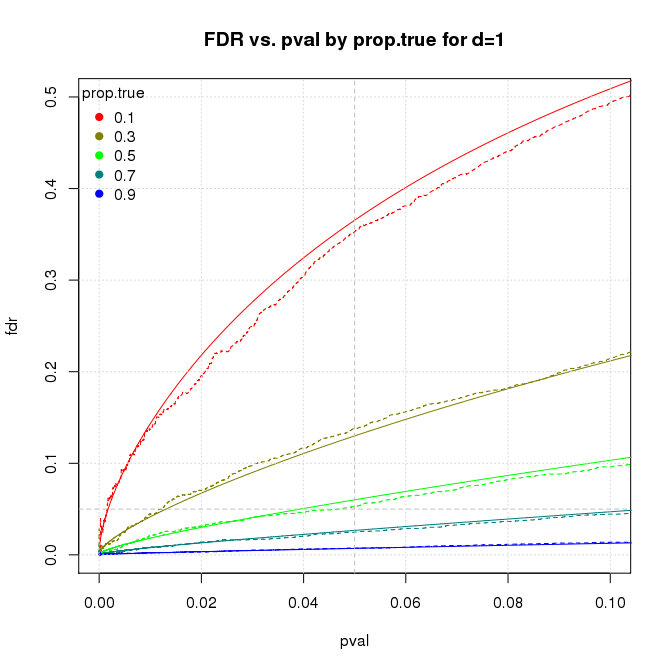# The 20% Statistician

A blog on statistics, methods, philosophy of science, and open science. Understanding 20% of statistics will improve 80% of your inferences.

## Monday, October 16, 2017

### Science-Wise False Discovery Rate Does Not Explain the Prevalence of Bad Science

Science-Wise False Discovery Rate Does Not Explain the Prevalence of Bad Science

This article explores the statistical concept of science-wise false discovery rate (SWFDR). Some authors use SWFDR and its complement, positive predictive value, to argue that most (or, at least, many) published scientific results must be wrong unless most hypotheses are a priori true. I disagree. While SWFDR is valid statistically, the real cause of bad science is “Publish or Perish”.

## Introduction

Is science broken? A lot of people seem to think so, including some esteemed statisticians. One line of reasoning uses the concepts of false discovery rate and its complement, positive predictive value, to argue that most (or, at least, many) published scientific results must be wrong unless most hypotheses are a priori true.

The false discovery rate (FDR) is the probability that a significant p-value indicates a false positive, or equivalently, the proportion of significant p-values that correspond to results without a real effect. The complement, positive predictive value (\(PPV=1-FDR\)) is the probability that a significant p-value indicates a true positive, or equivalently, the proportion of significant p-values that correspond to results with real effects.

I became interested in this topic after reading Felix Schönbrodt’s blog post, “What’s the probability that a significant p-value indicates a true effect?” and playing with his ShinyApp. Schönbrodt’s post led me to David Colquhoun’s paper, “An investigation of the false discovery rate and the misinterpretation of p-values” and blog posts by Daniel Lakens, “How can p = 0.05 lead to wrong conclusions 30% of the time with a 5% Type 1 error rate?” and Will Gervais, “Power Consequences”.

The term science-wise false discovery rate (SWFDR) is from Leah Jager and Jeffrey Leek’s paper, “An estimate of the science-wise false discovery rate and application to the top medical literature”. Earlier work includes Sholom Wacholder et al’s 2004 paper “Assessing the Probability That a Positive Report is False: An Approach for Molecular Epidemiology Studies” and John Ioannidis’s 2005 paper, “Why most published research findings are false”.

## Scenario

Being a programmer and not a statistician, I decided to write some R code to explore this topic on simulated data.

The program simulates a large number of problem instances representing published results, some of which are true and some false. The instances are very simple: I generate two groups of random numbers and use the t-test to assess the difference between their means. One group (the control group or simply group0) comes from a standard normal distribution with \(mean=0\). The other group (the treatment group or simply group1) is a little more involved:

• for true instances, I take numbers from a standard normal distribution with mean d (\(d>0\));
• for false instances, I use the same distribution as group0.

The parameter d is the effect size, aka Cohen’s d.

I use the t-test to compare the means of the groups and produce a p-value assessing whether both groups come from the same distribution.

The program does this thousands of times (drawing different random numbers each time, of course), collects the resulting p-values, and computes the FDR. The program repeats the procedure for a range of assumptions to determine the conditions under which most positive results are wrong.

For true instances, we expect the difference in means to be approximately d and for false ones to be approximately 0, but due to the vagaries of random sampling, this may not be so. If the actual difference in means is far from the expected value, the t-test may get it wrong, declaring a false instance to be positive and a true one to be negative. The goal is to see how often we get the wrong answer across a range of assumptions.

## Nomenclature

To reduce confusion, I will be obsessively consistent in my terminology.

• An instance is a single run of the simulation procedure.
• The terms positive and negative refer to the results of the t-test. A positive instance is one for which the t-test reports a significant p-value; a negative instance is the opposite. Obviously the distinction between positive and negative depends on the chosen significance level.
• true and false refer to the correct answers. A true instance is one where the treatment group (group1) is drawn from a distribution with \(mean=d\) (\(d>0\)). A false instance is the opposite: an instance where group1 is drawn from a distribution with \(mean=0\).
• empirical refers to results calculated from the simulated data, as opposed to theoretical which means results calculated using standard formulas.

The simulation parameters are

parameter meaning default
prop.true fraction of cases where there is a real effect `seq(.1,.9,by=.2)`
m number of iterations `1e4`
n sample size `16`
d standardized effect size (aka Cohen’s d) `c(.25,.50,.75,1,2)`
pwr power. if set, the program adjusts d to achieve power `NA`
sig.level significance level for power calculations when pwr is set `0.05`
pval.plot p-values for which we plot results `c(.001,.01,.03,.05,.1)`

## Results

The simulation procedure with default parameters produces four graphs similar to the ones below.